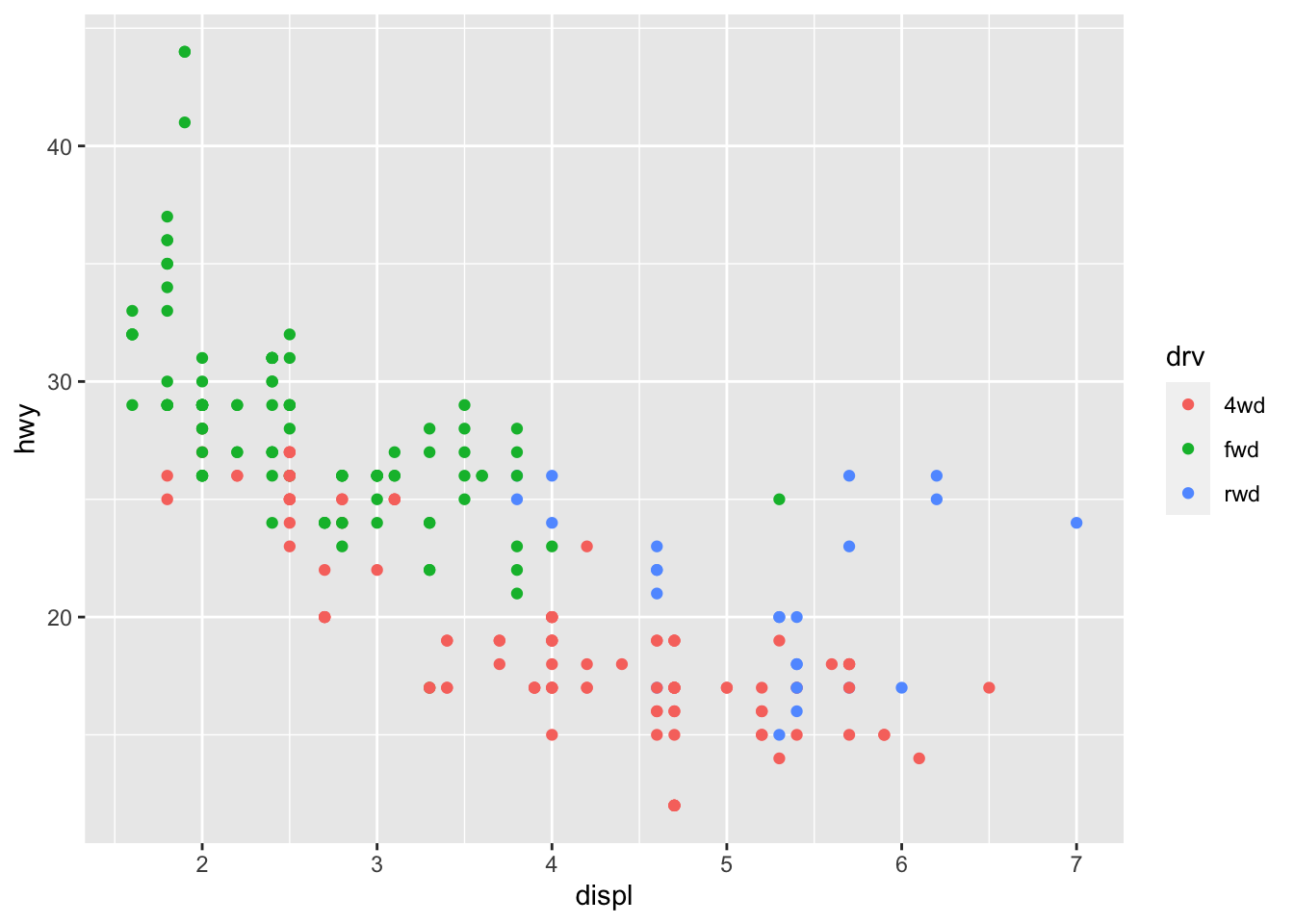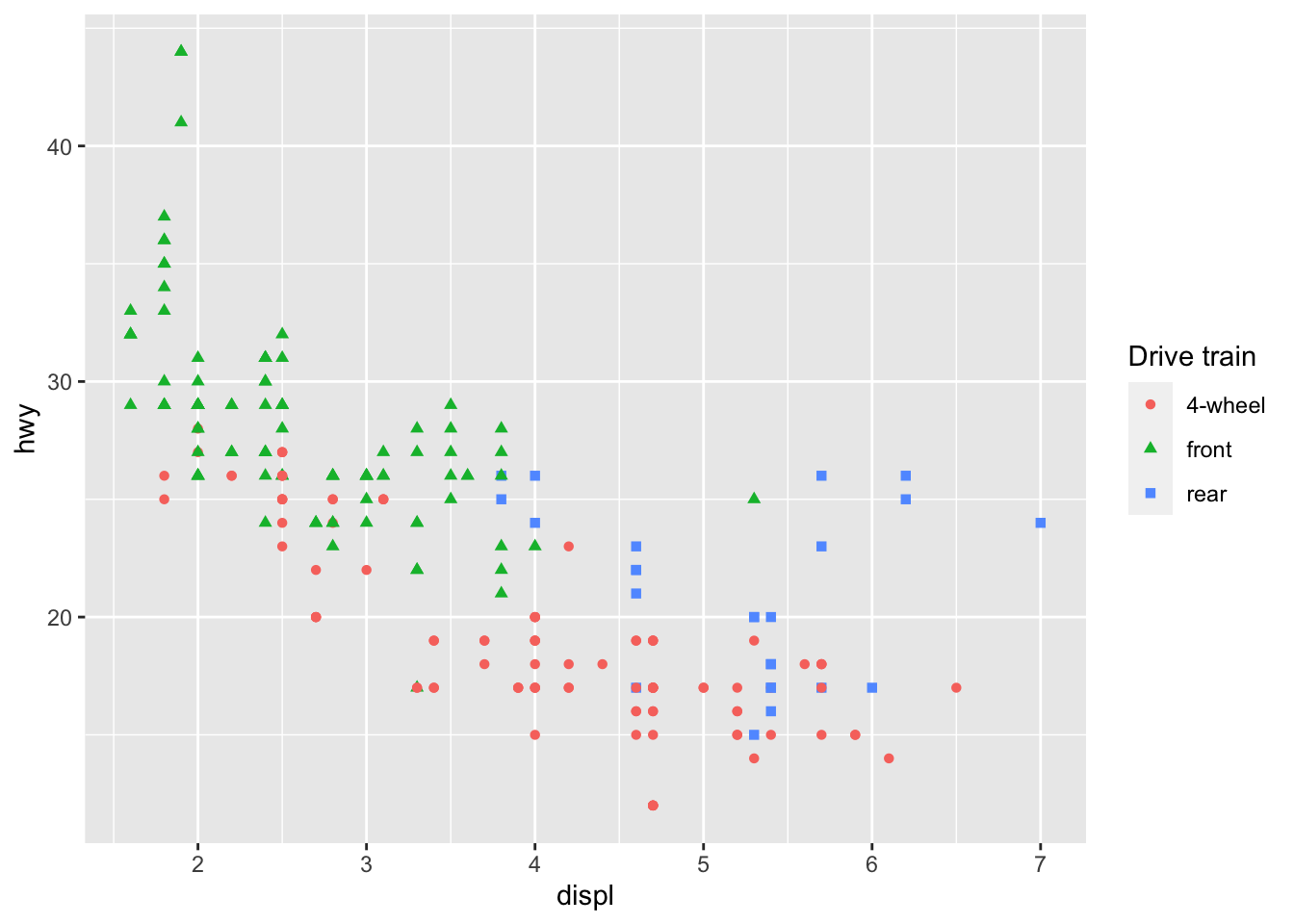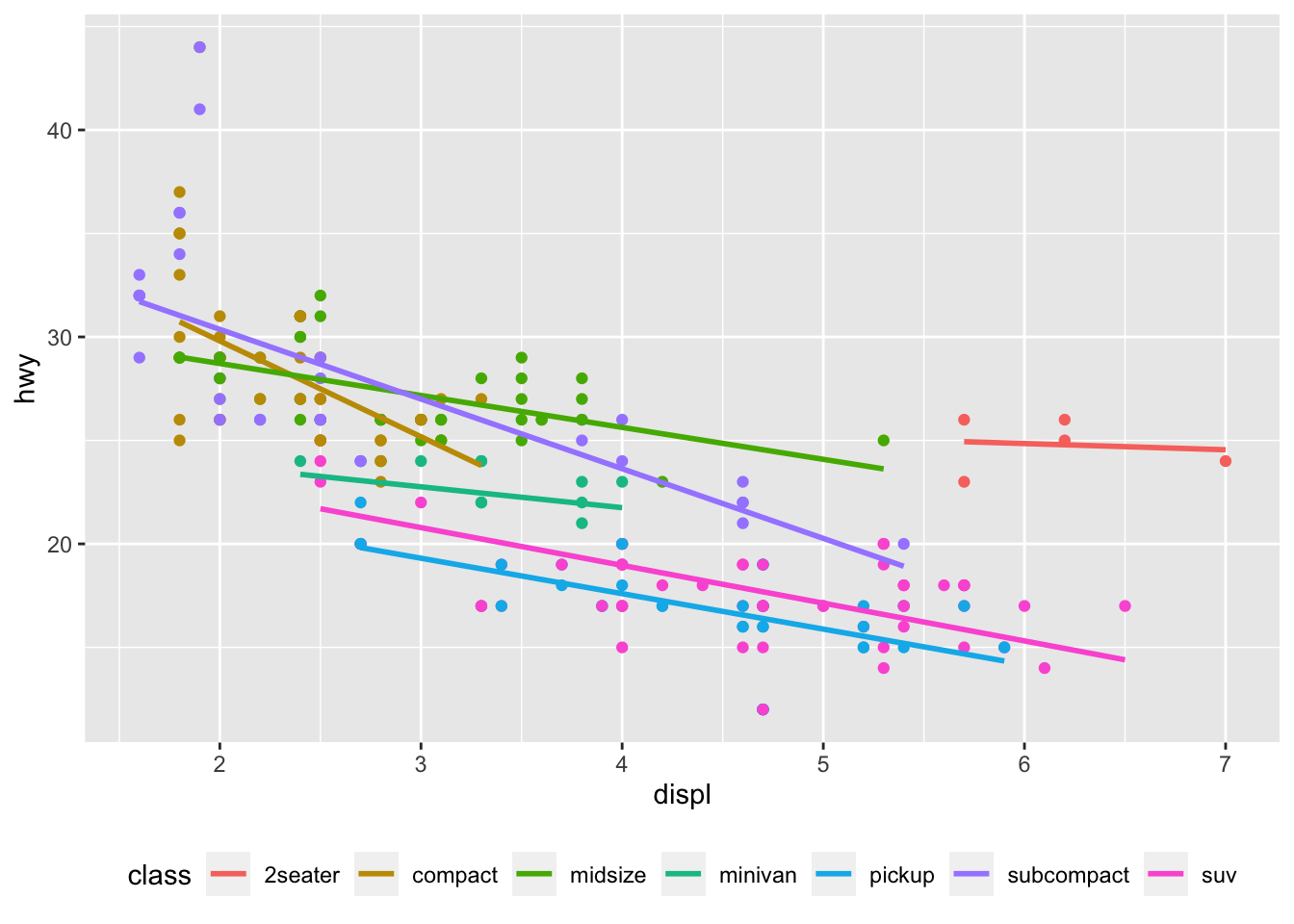# 11 Colour scales and legends

## 11.1 Exercises

``````drv_labels <- c("4" = "4wd", "f" = "fwd", "r" = "rwd")

ggplot(mpg, aes(displ, hwy)) +
geom_point(aes(colour = drv)) +
scale_colour_discrete(labels = drv_labels)``````• We store the labels inside `drv_labels` and use it in `scale_colour_discrete()`

## 11.2 Exercises

1. How do you make legends appear to the left of the plot? `-`theme(legend.position = “left”)`make legends appear to the left of the plot. - Other options:`theme(legend.position = “right”)`,`theme(legend.position = “bottom”)`, and`theme(legend.position = “none”)`

2. What’s gone wrong with this plot? How could you fix it?

• There are two separate legends for the same variable (`drv`). We need to combine these two legends into one. To do this, both `color` and `shape` need to be given shape specifications.
``````ggplot(mpg, aes(displ, hwy)) +
geom_point(aes(colour = drv, shape = drv)) +
scale_colour_discrete("Drive train",
breaks = c("4", "f", "r"),
labels = c("4-wheel", "front", "rear")) +
scale_shape_discrete("Drive train",
breaks = c("4", "f", "r"),
labels = c("4-wheel", "front", "rear"))``````3.

``````ggplot(mpg, aes(displ, hwy, colour = class)) +
geom_point(show.legend = FALSE) +
geom_smooth(method = "lm", se = FALSE) +
theme(legend.position = "bottom") +
guides(colour = guide_legend(nrow = 1))
#> `geom_smooth()` using formula 'y ~ x'``````Note: The answers to these “recreate the code for this plot” questions are provided in the source code of the book.